# JP2010028607A - Image processing apparatus, image capturing apparatus and image processing mehtod - Google Patents

Image processing apparatus, image capturing apparatus and image processing mehtod Download PDF

## Info

Publication number
JP2010028607A
JP2010028607A JP2008189413A JP2008189413A JP2010028607A JP 2010028607 A JP2010028607 A JP 2010028607A JP 2008189413 A JP2008189413 A JP 2008189413A JP 2008189413 A JP2008189413 A JP 2008189413A JP 2010028607 A JP2010028607 A JP 2010028607A
Authority
JP
Japan
Prior art keywords
image
motion
motion vector
input image
motion information
Prior art date
Legal status (The legal status is an assumption and is not a legal conclusion. Google has not performed a legal analysis and makes no representation as to the accuracy of the status listed.)
Pending
Application number
JP2008189413A
Other languages
Japanese (ja)
Inventor
Sukeki Akakabe
Yukio Mori

Original Assignee
Sanyo Electric Co Ltd

Priority date (The priority date is an assumption and is not a legal conclusion. Google has not performed a legal analysis and makes no representation as to the accuracy of the date listed.)
Filing date
Publication date
Application filed by Sanyo Electric Co Ltd, 三洋電機株式会社 filed Critical Sanyo Electric Co Ltd
Priority to JP2008189413A priority Critical patent/JP2010028607A/en
Publication of JP2010028607A publication Critical patent/JP2010028607A/en
Application status is Pending legal-status Critical

## Images

•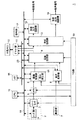•••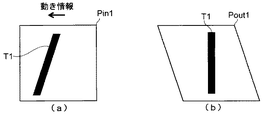•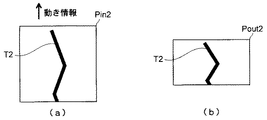•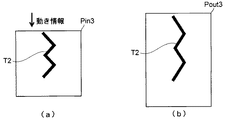•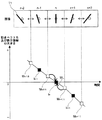•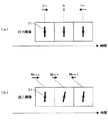•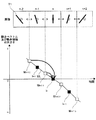•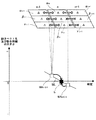•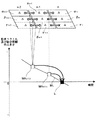•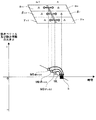•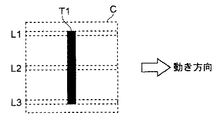••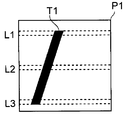•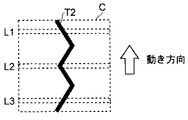•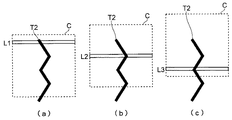•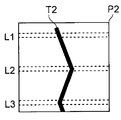•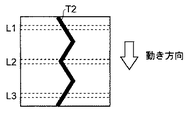•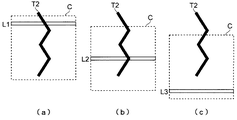•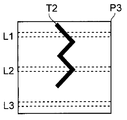## Abstract

<P>PROBLEM TO BE SOLVED: To provide an image processing apparatus capable of accurately correcting a strain, a method for processing an image and an image capturing apparatus with the image processing apparatus. <P>SOLUTION: When the strain of n-th image is corrected, motion information I<SB>n</SB>indicating a motion for the exposure period of n-th image is computed by using a motion vector M<SB>n-0.5</SB>determined from n-1th image and n-th image and the motion vector M<SB>n+0.5</SB>determined from n-th image and n+1th image. Consequently, the strain caused by the motion generated during n-th exposure period can be corrected accurately. <P>COPYRIGHT: (C)2010,JPO&INPIT

## Description

The present invention relates to an image processing apparatus that processes an input image and an imaging apparatus equipped with the image processing apparatus. The present invention also relates to an image processing method for processing an input image.

2. Description of the Related Art In recent years, imaging devices that create an image that suppresses blurring caused by movement of an imaging device or a subject (an imaging target such as a person) have been widely used. Some of such imaging devices are equipped with an image processing device that detects a blur from an input image and generates and outputs an image in which the blur is suppressed. In this way, if the configuration is configured to suppress blurring by image processing, a sensor that detects the movement of the imaging device, a device that suppresses the movement of the imaging device, and the like are unnecessary, and thus the imaging device is reduced in size and weight. Is possible.

Further, as one of the above-mentioned blurs, there is a so-called focal plane distortion (hereinafter sometimes simply referred to as “distortion”). The focal plane distortion is, for example, distortion that may occur in a CMOS (Complementary Metal Oxide Semiconductor) image sensor in which exposure and charge reading are controlled for each pixel column. In particular, this is distortion that occurs because the exposure period differs for each pixel column.

Specific examples of focal plane distortion are shown in FIGS. 13 to 15 are schematic diagrams for explaining the focal plane distortion. FIG. 13 is a schematic diagram illustrating a subject immediately before imaging, an imaging region, and a movement direction thereof, and FIG. 14 is a schematic diagram illustrating a positional relationship between the subject and the imaging region in an exposure period of each pixel column. FIG. 15 is a schematic diagram showing an image including distortion. It is assumed that the subject T1 is stationary. Further, it is assumed that exposure is started in the order of the pixel column L1, the pixel column L2, and the pixel column L3 in the representative pixel columns L1 to L3 shown in FIG. In other words, in the direction perpendicular to the pixel column (hereinafter referred to as the vertical direction), the upper pixel column has an earlier exposure period, and the lower one has a shorter exposure period. Further, the imaging region C moves at a constant speed along the movement direction shown in FIG. 13 (the direction parallel to the pixel rows L1 to L3 (hereinafter referred to as the horizontal direction) and the right direction in the drawing). And At this time, the imaging apparatus translates from left to right in the horizontal direction, or rotates from left to right in the pan direction.

When the imaging region C moves as described above, as shown in FIGS. 14A to 14C, the imaging operation starts from the exposure of the pixel row L1 with the earlier exposure period to the exposure of the pixel row L3 with the later exposure period. As it progresses, the position of the subject T1 with respect to the imaging region C moves in the direction opposite to the movement direction (left direction in the figure). Therefore, as shown in FIG. 15, in the obtained image P <b> 1, the lower pixel column is shifted from the upper pixel column in the direction opposite to the movement direction. That is, the image P1 is distorted with respect to the horizontal direction, which is a direction parallel to the movement direction.

This focal plane distortion also occurs when the imaging region C moves in the vertical direction. For example, FIGS. 16 to 18 show a case where the imaging region C moves upward. FIG. 16 is a schematic diagram illustrating a subject immediately before imaging, an imaging region, and a moving direction thereof, and FIG. 17 is a schematic diagram illustrating a positional relationship between the subject and the imaging region in an exposure period of each pixel column. FIG. 18 is a schematic diagram showing an image including distortion. It is assumed that the conditions are the same as those in the cases illustrated in FIGS. 13 to 15, and the movement directions of the subject T2 and the imaging region C are different. The imaging region C is assumed to move at a constant speed along the movement direction (vertical direction, upward in the figure) shown in FIG. At this time, the imaging device translates from the bottom to the top in the vertical direction, or rotates from the bottom to the top in the tilt direction.

When the imaging region C moves as described above, as shown in FIGS. 17A to 17C, the imaging operation starts from the exposure of the pixel row L1 with the earlier exposure period to the exposure of the pixel row L3 with the later exposure period. As the movement proceeds, the position of the subject T2 with respect to the imaging region C moves in the direction opposite to the movement direction (downward in the figure). Therefore, as shown in FIG. 18, the obtained image P2 appears to be expanded in the vertical direction. That is, the image P2 is distorted with respect to the vertical direction, which is a direction parallel to the movement direction.

On the other hand, FIGS. 19 to 21 show a case where the imaging region C moves downward. FIG. 19 is a schematic diagram illustrating a subject immediately before imaging, an imaging region, and a moving direction thereof, and FIG. 20 is a schematic diagram illustrating a positional relationship between the subject and the imaging region in an exposure period of each pixel column. FIG. 21 is a schematic diagram showing an image including distortion. It is assumed that the conditions are the same as those shown in FIGS. 16 to 18, and only the movement direction of the imaging region C is different. The imaging area C is assumed to move at a constant speed along the movement direction (vertical direction, downward in the figure) shown in FIG. At this time, the imaging apparatus translates from top to bottom in the vertical direction or rotates from top to bottom in the tilt direction.

When the imaging apparatus moves as described above, as shown in FIGS. 20A to 20C, the imaging operation proceeds from the exposure of the pixel column L1 with the earlier exposure period to the exposure of the pixel column L3 with the later exposure period. Accordingly, the position of the subject T2 with respect to the imaging region C moves in the direction opposite to the movement direction (upward in the figure). Therefore, as shown in FIG. 21, the obtained image P3 is compressed in the vertical direction. That is, the image P3 is distorted with respect to the vertical direction, which is a direction parallel to the movement direction.

Further, if the movement direction is an oblique direction (a direction between the horizontal direction and the vertical direction), distortion caused by movement in both the horizontal direction and the vertical direction occurs. In the above example, the case where the imaging apparatus moves and the subjects T1 and T2 are stationary has been described. However, the focal plane distortion can similarly occur when the subject moves.

As a method for reducing such focal plane distortion by image processing, for example, Patent Document 1 proposes a method for reducing focal plane distortion based on a motion vector between two images input in succession. Yes. Specifically, the focal plane distortion of the subsequent image is reduced by calculating the distortion of the subsequent image using a motion vector obtained from the previous image and the subsequent image, and applying image processing that cancels this distortion to the subsequent image. To do.
JP 2007-208580 A

However, in the above-described method, if the previous image is not an image without distortion (an image without movement during exposure), there is a possibility that erroneous image processing is performed on the subsequent image. Specifically, for example, when the previous image is an image with distortion and the subsequent image is an image without distortion. In this case, there is a possibility of erroneously recognizing that a motion has occurred during exposure of the subsequent image from the obtained motion vector between the previous image and the subsequent image, and performing an incorrect correction on the subsequent image. Therefore, it becomes difficult to perform distortion correction with high accuracy.

SUMMARY An advantage of some aspects of the invention is that it provides an image processing apparatus and an image processing method capable of accurately correcting distortion. Moreover, it aims at providing the imaging device provided with this image processing apparatus.

In order to achieve the above object, an image processing apparatus according to the present invention corrects distortion of an input image that is one of input images while inputting a plurality of images having different exposure periods at the time of imaging. In the output image processing apparatus, a motion vector calculation unit that compares two input images and calculates a motion vector indicating a motion between the two images, and the motion vector output from the motion vector calculation unit A motion information calculation unit that calculates motion information indicating a motion of an exposure period of the input image based on the input image, and the input image is input and based on the motion information output from the motion information calculation unit And a correction processing unit that corrects distortion of the input image and outputs the output image as an output image.

Further, in the image processing apparatus having the above-described configuration, the image is picked up so that the exposure period is earlier in the upper pixel row and the exposure period is slower in the lower pixel row. The distortion may be corrected for each pixel column of the input image based on the motion information.

In the image processing apparatus having the above configuration, the correction processing unit may correct the reading position for each pixel column of the input image based on the motion information and output the corrected output image as the output image.

For example, when correcting the movement in a direction substantially perpendicular to the vertical direction (direction substantially parallel to the pixel column), the reading start position of the lower pixel column is moved more than the reading start position of the upper pixel column. It corrects so that it may be in the direction opposite to the direction. When correcting the movement in the direction substantially equal to the upward direction, correction is performed so that the readout position of each pixel column approaches the center pixel column. At this time, thinning out of pixels and addition reading may be performed. Further, when correcting the movement in the direction substantially equal to the downward direction, correction is performed so that the reading position of each pixel column is away from the center pixel column. At this time, the pixel rows to be read may be interpolated.

In the image processing apparatus having the above configuration, the motion vector calculation unit calculates at least one motion vector calculated by using at least one image having an exposure period earlier than the input image and an image having an exposure period later than the input image. At least one motion vector to be calculated using the motion vector, and the motion information calculation unit calculates the motion information using at least two motion vectors calculated by the motion vector calculation unit. It doesn't matter.

If comprised in this way, when calculating the motion information of an input image, it becomes possible to reflect the image before and behind an input image. Therefore, it is possible to obtain the motion information of the input image with high accuracy.

In the image processing apparatus having the above-described configuration, the motion vector calculation unit includes at least two motion vectors calculated from the input image and two images selected from images having an exposure period earlier than the input image. The motion information calculation unit may calculate the motion information using at least two motion vectors calculated by the motion vector calculation unit.

If comprised in this way, when calculating the motion information of an input image, the image whose exposure period is later than an input image becomes unnecessary. Therefore, it is possible to suppress generation of an output image after an image subsequent to the input image is input. Therefore, it is possible to quickly generate an output image.

In the image processing apparatus having the above-described configuration, the image is divided into two or more middle regions in the vertical direction, and the exposure time of the upper middle region is earlier and the lower middle region of the image is exposed. The image is picked up so that the period is delayed, and the motion vector calculation unit compares the two input images for each middle region and calculates a middle region motion vector indicating the motion between the middle regions to be compared. In addition, the motion vector may be calculated based on the middle region motion vector calculated from the two images.

In the image processing apparatus having the above-described configuration, the image is divided into two or more middle regions in the vertical direction, and the exposure time of the upper middle region is earlier and the lower middle region of the image is exposed. The image is picked up so that the period is delayed, and the motion vector calculation unit compares the two input images for each middle region and calculates a middle region motion vector indicating the motion between the middle regions to be compared. In addition, at least one of the middle region motion vectors calculated from the two images may be used as the motion vector.

In the image processing apparatus having the above-described configuration, when the motion vector calculation unit obtains an unsuitable result when calculating the middle region motion vector, the middle region motion vector may be excluded. .

Here, the inappropriate result is, for example, a case where a middle region motion vector is not obtained even when the middle regions of two images are compared, or a case where the reliability of the middle region motion vector is low. In particular, it is assumed that a calculation result that may deteriorate accuracy when calculating a motion vector or motion information is an inappropriate result.

If comprised in this way, it will become possible to obtain | require a motion vector and motion information excluding the result which becomes unsuitable. Therefore, it is possible to obtain the motion information of the input image with high accuracy.

The imaging device of the present invention includes an imaging unit that captures an image and creates an image, and any one of the image processing devices described above, and the imaging unit creates a plurality of images with different exposure periods and Input to an image processing apparatus, and the image processing apparatus corrects distortion of an input image, which is one of the input images, and outputs it as an output image.

The image processing method of the present invention is an image processing method for correcting and outputting distortion included in an input image that is one of images based on a plurality of images having different exposure periods at the time of imaging. A first step of comparing images and calculating a motion vector indicating a motion between the two images, and a motion of the exposure period of the input image based on the motion vector calculated in the first step A second step of calculating motion information; and a third step of correcting distortion of the input image based on the motion information calculated in the second step and outputting the output image as an output image. To do.

With the configuration of the present invention, the motion information indicating the motion of the exposure period of the input image is calculated from the motion vector indicating the motion between the two images, and the distortion of the input image is corrected based on this motion information. . For this reason, it is possible to suppress misperception of the movement of the exposure period of the input image, and it is possible to correct the input image with high accuracy.

Embodiments of an image processing apparatus, an image processing method, and an imaging apparatus according to the present invention will be described below with reference to the drawings. First, the basic configuration and basic operation of the imaging apparatus will be described.

<Imaging device>
(Basic configuration)
First, the basic configuration of the imaging apparatus will be described with reference to FIG. FIG. 1 is a block diagram illustrating a basic configuration of an imaging apparatus according to an embodiment of the present invention.

As shown in FIG. 1, an imaging apparatus 1 forms an image sensor 3 including a solid-state imaging device such as a CMOS sensor that converts incident light into an electrical signal, and an optical image of a subject on the image sensor 3 and a light amount. And an imaging unit 2 including a lens unit 4 that performs adjustment such as the above.

Further, the imaging device 1 performs gradation correction on an AFE (Analog Front End) 5 that converts an image signal that is an analog signal output from the image sensor 3 into a digital signal, and a digital image signal output from the AFE 5. An image processing unit 6 that performs various image processing such as processing, a sound collecting unit 7 that converts input sound into an electric signal, and an analog sound signal output from the sound collecting unit 7 is converted into a digital signal and sound MPEG (Moving Picture Experts Group) for each of the audio processing unit 8 that performs various audio processing such as noise removal on the signal, and the image signal output from the image processing unit 6 and the audio signal output from the audio processing unit 8 Pressure for still images such as JPEG (Joint Photographic Experts Group) compression method is applied to the image signal that is subjected to compression encoding processing for moving images such as a compression method or output from the image processing unit 6 A compression processing unit 9 that performs compression coding processing, an external memory 10 that records a compression-coded signal that has been compression-coded by the compression processing unit 9, and a recording and reading of the compression-coded signal in the external memory 10 And a decompression processing unit 12 that decompresses and decodes the compressed encoded signal read from the external memory 10 in the driver unit 11.

The imaging device 1 also includes an image output circuit unit 13 that converts an image signal obtained by decoding by the expansion processing unit 12 into an analog signal for display on a display device (not shown) such as a display, and the expansion processing unit 12. And an audio output circuit unit 14 for converting the audio signal obtained by decoding into an analog signal for reproduction by a reproduction device (not shown) such as a speaker.

The imaging apparatus 1 also stores a CPU (Central Processing Unit) 15 that controls the entire operation of the imaging apparatus 1 and a memory 16 that stores each program for performing each process and temporarily stores data when the program is executed. And a timing generator (TG) unit that outputs a timing control signal for matching the operation timing of each unit, and an operation unit 17 to which an instruction from a user is input, such as a button for starting imaging and a button for adjusting imaging conditions. 18, a bus line 19 for exchanging data between the CPU 15 and each block, and a bus line 20 for exchanging data between the memory 16 and each block.

The image processing unit 6 also includes a distortion correction unit 61 that corrects and outputs distortion included in the input image signal. Details of the configuration of the distortion correction unit 61 will be described later.

Note that the imaging apparatus 1 that can generate image signals of moving images and still images is shown as an example, but the imaging apparatus 1 may be capable of generating only image signals of still images. In this case, a configuration without the sound collection unit 7, the audio processing unit 8, the audio output circuit unit 14, and the like may be used.

The external memory 10 may be anything as long as it can record image signals and audio signals. For example, a semiconductor memory such as an SD (Secure Digital) card, an optical disk such as a DVD, a magnetic disk such as a hard disk, or the like can be used as the external memory 10. Further, the external memory 10 may be detachable from the imaging device 1.

(basic action)
Next, the basic operation of the imaging apparatus 1 will be described with reference to FIG. First, the imaging device 1 acquires an image signal that is an electrical signal by photoelectrically converting light incident from the lens unit 4 in the image sensor 3. Then, the image sensor 3 outputs an image signal to the AFE 5 at a predetermined timing in synchronization with the timing control signal input from the TG unit 18.

Then, the image signal converted from the analog signal to the digital signal by the AFE 5 is input to the image processing unit 6. The image processing unit 6 converts an input image signal having R (red), G (green), and B (blue) components into an image signal having luminance signal (Y) and color difference signals (U, V). In addition, various image processing such as gradation correction and contour enhancement is performed. The memory 16 operates as a frame memory, and temporarily holds an image signal when the image processing unit 6 performs processing.

At this time, based on the image signal input to the image processing unit 6, the lens unit 4 adjusts the position of various lenses to adjust the focus, or adjusts the opening of the diaphragm to adjust the exposure. It is done. This adjustment of focus and exposure is automatically performed based on a predetermined program so as to be in an optimum state, or manually performed based on a user instruction.

In addition, the image processing unit 6 corrects image distortion in the distortion correction unit 61. Details of the operation of the distortion correction unit 61 will be described later.

When creating an image signal of a moving image, the sound collecting unit 7 collects sound. An audio signal collected by the sound collection unit 7 and converted into an electric signal is input to the audio processing unit 8. The sound processing unit 8 converts an input sound signal into a digital signal and performs various sound processing such as noise removal and sound signal intensity control. The image signal output from the image processing unit 6 and the audio signal output from the audio processing unit 8 are both input to the compression processing unit 9 and compressed by the compression processing unit 9 using a predetermined compression method. At this time, the image signal and the audio signal are associated with each other in time, and the image and the sound are not shifted during reproduction. The compressed encoded signal output from the compression processing unit 9 is recorded in the external memory 10 via the driver unit 11.

On the other hand, when creating an image signal of a still image, the image signal output from the image processing unit 6 is input to the compression processing unit 9 and compressed by the compression processing unit 9 using a predetermined compression method. The compressed encoded signal output from the compression processing unit 9 is recorded in the external memory 10 via the driver unit 11.

The compressed encoded signal of the moving image recorded in the external memory 10 is read out to the decompression processing unit 12 based on a user instruction. The decompression processing unit 12 decompresses and decodes the compressed encoded signal, and generates an image signal and an audio signal. The image signal is output to the image output circuit unit 13 and the audio signal is output to the audio output circuit unit 14. Then, in the image output circuit unit 13 and the audio output circuit unit 14, it is converted into a format that can be reproduced by a display device or a speaker and output.

On the other hand, a still image compression-encoded signal recorded in the external memory 10 is input to the decompression processing unit 12 to generate an image signal. The image signal is output to the image output circuit unit 13, converted into a format that can be reproduced by the display device in the image output circuit unit 13, and output.

The display device and the speaker may be integrated with the imaging device 1 or may be separated and connected to a terminal provided in the imaging device 1 with a cable or the like. It does not matter.

Further, in a so-called preview mode in which the user confirms an image displayed on a display device or the like without recording an image signal, the image output circuit without compressing the image signal output from the image processing unit 6 It may be output to the unit 13. In addition, when recording an image signal, the image signal may be output to a display device or the like via the image output circuit unit 13 in parallel with the operation of compressing by the compression processing unit 9 and recording in the external memory 10. I do not care.

<Distortion correction unit>
Next, the distortion correction unit 61 provided in the image processing unit 6 (image processing apparatus) illustrated in FIG. 1 will be described in detail. In the following description, the image signal of each image processed by the distortion correction unit 61 is expressed as an image for the sake of concrete explanation. In addition, an image to be subjected to distortion correction is an “input image”, and an image after the distortion correction is performed is an “output image”.

In the following description, a direction parallel to the pixel column, which is a unit of exposure and charge readout control, is defined as a horizontal direction of an image. The direction perpendicular to the pixel column is defined as the vertical direction of the image. Further, it is particularly problematic that the exposure start time is different for each pixel column (exposure period is different), and the difference in the exposure period for each pixel in the pixel column is negligible. In addition, when capturing one image, among the pixel columns aligned in the vertical direction, the upper pixel column starts exposure earlier (exposure period is earlier), and the lower pixel column starts exposure. Is slow (exposure period is slow).

Further, the image processing unit 6 provided in the imaging apparatus 1 of the present embodiment can process both a moving image signal and a still image signal. For this reason, the above-described image represents both a moving image signal and a still image signal. It is assumed that a plurality of image signals are input even if one still image signal is output.

(Basic configuration)
First, the basic configuration of the distortion correction unit 61 will be described with reference to FIG. FIG. 2 is a block diagram illustrating a basic configuration of a distortion correction unit provided in the imaging apparatus according to the embodiment of the present invention. As illustrated in FIG. 2, the distortion correction unit 61 includes a motion vector calculation unit 62 that calculates a motion vector from two input images (a first image and a second image whose exposure period is later than the first image). A motion information calculation unit 63 that calculates motion information using the motion vector calculated by the motion vector calculation unit 62, and a correction process that corrects distortion of the input image based on the motion information calculated by the motion information calculation unit 63 Unit 64.

The motion vector is obtained by comparing the first image and the second image. In addition, the obtained motion vector indicates a motion between the first image and the second image (including the motion of the imaging device and the motion of the subject, hereinafter simply expressed as “motion”). Specifically, for example, it represents the direction and magnitude of movement from the start of the exposure period of the first image to the end of the exposure period of the second image.

The motion information indicates the motion of the input image during the exposure period, and is calculated using a motion vector. Similarly to the motion vector, the motion information represents the direction and magnitude of the motion. Therefore, it is possible to interpret the motion information as a kind of motion vector.

The basic operation of the distortion correction unit 61 will be described with reference to FIG. FIG. 3 is a flowchart illustrating the basic operation of the distortion correction unit provided in the imaging apparatus according to the embodiment of the present invention. FIG. 3 is a flowchart showing processing for correcting distortion of one input image. Therefore, in the case of creating a moving image signal, the operations shown in FIG. 3 are sequentially performed.

As shown in FIG. 3, first, the motion vector calculation unit 62 calculates a motion vector between both images based on the input first image and second image (STEP 1). Thereby, a motion vector between the first image and the second image is obtained.

Next, the motion information calculation unit 62 calculates the motion information of the input image using the motion vector calculated in STEP 1 (STEP 2). At this time, the motion information calculation unit 62 calculates motion information with reference to at least two motion vectors. For this reason, the motion information calculation unit 62 is configured to be able to temporarily store or refer to motion vectors input in the past.

Then, the correction processing unit 64 performs correction on the input image based on the motion information obtained in STEP 2 to generate an output image (STEP 3). In particular, the correction processing is performed by performing correction on the input image so as to cancel the movement indicated by the movement information. The correction that cancels the motion is, for example, correction that gives the input image distortion generated in the image when the motion in the opposite direction to the motion indicated by the motion information occurs.

Specifically, for example, the correction can be performed by using a method of controlling the reading of the image signal of the input image held in the frame memory or the line memory for each pixel column. A specific example of this correction method will be described with reference to FIGS. Each of FIGS. 4 to 6 is a schematic diagram illustrating an example of the correction process. 4 (a), 5 (a) and 6 (a) show an example of an input image and motion information. FIG. 4 (b), FIG. 5 (b) and FIG. 6 (b) Shows an example of an output image.

FIG. 4 shows a case where motion is detected in a direction substantially parallel to the horizontal direction. In particular, the case where a leftward movement is detected is shown. Note that the input image Pin1 corresponds to the image P1 in FIG. Also, the output image Pout1 in FIG. 4B shows a range where the read image signal exists surrounded by a solid line.

When the motion information as described above is output, the correction processing unit 64 reads the upper pixel column to the left and the lower pixel column to the left as shown in the output image Pout1 of FIG. Control is performed so that the position is on the right. That is, the reading position is controlled so that the lower pixel column is shifted in the direction opposite to the direction of motion indicated by the motion information from the upper pixel column.

On the other hand, in contrast to FIG. 4A, when motion in the right direction is detected, control is performed so that the readout position is on the right side of the upper pixel column and the readout position is on the left side of the lower pixel column. .

FIG. 5 shows a case where motion is detected in a direction substantially parallel to the vertical direction. In particular, the case where an upward movement is detected is shown. Note that the input image Pin2 corresponds to the image P2 in FIG. Further, the output image Pout2 in FIG. 5B shows a portion where the read image signal exists surrounded by a solid line.

As described above, when an upward movement occurs, the input image Pin2 is expanded in the vertical direction. Therefore, the correction processing unit 64 performs correction for compression in the vertical direction on the input image Pin2 to obtain an output image Pout2.

For example, reading is performed so that the position of the image signal of each pixel column of the input image Pin2 is a position close to the center pixel column of the output image Pout2. At this time, thinning-out reading may be performed without reading out an image signal of a predetermined pixel column, or addition reading may be performed in which image signals of predetermined adjacent pixel columns are added and read as an image signal of one pixel column. Absent. And the output image Pout2 which compressed the input image Pin2 as shown in FIG.5 (b) with respect to the orthogonal | vertical direction by the above methods is obtained.

On the other hand, FIG. 6 shows a case where a downward movement is detected. Note that the input image Pin3 corresponds to the image P3 in FIG. In addition, the output image Pout3 in FIG. 6B is a portion in which the read image signal exists is surrounded by a solid line.

As described above, when a downward movement occurs, the input image Pin3 is compressed in the vertical direction. Therefore, the correction processing unit 64 performs correction that extends in the vertical direction on the input image Pin3 to obtain an output image Pout3.

For example, reading is performed so that the image signal of each pixel column of the input image Pin3 is separated from the center pixel column of the output image Pout3. At this time, interpolation reading may be performed in which image signals between the pixel columns are interpolated using image signals of predetermined adjacent pixel columns, and an image signal obtained by the interpolation is read as an image signal between the pixel columns. Absent. Then, an output image Pout3 obtained by extending the input image Pin3 as shown in FIG. 6B with respect to the vertical direction is obtained by the method described above.

With the configuration as described above, motion information indicating the motion of the exposure period of the input image is calculated from the motion vector indicating the motion between the two images, and distortion of the input image is corrected based on the motion information. Become. Therefore, it is possible to accurately correct the movement of the input image during the exposure period.

In particular, for example, when there is distortion in the previous image captured immediately before the input image and there is no distortion in the input image, the motion vector obtained from the previous image and the input image indicates that there is motion, The motion information of the input image indicates that there is no motion. For this reason, it is possible to suppress misperception of the movement that occurs during the exposure period of the input image, and it is possible to suppress erroneous correction of the input image.

Note that an image obtained by further correcting the output images Pout1 to Pout3 in FIGS. 4B, 5B, and 6B may be used as the output image. Specifically, a predetermined rectangular area may be set for the output images Pout1 to Pout3, and an output image may be generated using an image signal in the rectangular area. Furthermore, the rectangular area may be reduced (for example, pixel addition or thinning), or may be enlarged (for example, interpolation between pixels). Further, the rectangular area may be set based on the motion information, or may be set based on the position where the subjects T1 and T2 exist.

4 to 6, the distortion correction methods are separately described in the horizontal direction and the vertical direction for convenience of description. However, these can be performed simultaneously. In particular, when motion in an oblique direction is detected, distortion is generated in the horizontal direction and the vertical direction of the input image. Therefore, it is preferable to perform correction in both directions. At this time, the correction for the horizontal direction and the correction for the vertical direction may be performed in order or simultaneously.

In FIG. 2, the first image, the second image, and the input image are described as different images. However, the first image and the input image are the same, or the second image and the input image are the same. It may be a thing.

The configuration of each embodiment of the distortion correction unit 61 described below is the same. In particular, the configuration is the same as the configuration shown in FIG. 2 described as the basic configuration of the distortion correction unit 61. Therefore, in the following description of each embodiment of the distortion correction unit 61, the configuration of each embodiment is assumed to be the same as that shown in FIG.

(First embodiment)
Next, a first embodiment of the distortion correction unit 61 will be described with reference to the drawings. FIG. 7 is a graph showing an operation example of the distortion correction unit of the first embodiment. Specifically, the case where n-2th, n−1th, nth, n + 1th, and n + 2th images are sequentially generated and input to the distortion correction unit 61 is illustrated. The exposure period of each image is delayed in the order of n-2, n-1, n, n + 1, and n + 2 (where n is a natural number of 3 or more). In the following description, when referring to the previous image and the subsequent image, the front and back in the above order are indicated. In the following description, an example in which a horizontal movement of an image occurs will be described.

FIG. 7 is a graph showing the motion vector and the size of the motion information obtained from each of the images, and the time when the motion indicated by the motion vector and the motion information occurs. Note that the vertical axis indicates the magnitude of the motion vector and the motion information in the horizontal direction, and the horizontal axis indicates time. If the magnitude of the motion vector is positive, it indicates that a rightward movement has occurred. On the other hand, if the magnitude of the motion vector is negative, it indicates that a leftward movement has occurred. In addition, the average time of the exposure period of each entire image is shown as a representative of the exposure period as the image average exposure time of the image (white circle in the image in FIG. 7), and for each pixel column of each image The difference in exposure period is not shown.

The motion vector calculation unit 62 in the distortion correction unit 61 of this embodiment calculates a motion vector (white square in FIG. 7) between the two images. This motion vector is calculated from the result of comparing the entire two images. Therefore, for example, it can be considered to indicate the movement of the average time of the exposure period of two images (that is, the average time of the image average exposure time of two images).

The motion vector calculation unit 62 can obtain a motion vector using various methods such as a block matching method, a representative point matching method, and a gradient method, and methods other than those described above may be used. The image is divided into h areas in the horizontal direction and i areas in the vertical direction to form h × i small areas. For each small area, for example, the above method is applied to calculate a small area motion vector, and these are averaged. It is also possible to obtain a motion vector by converting (where h and i are natural numbers).

The motion information calculation unit 63 uses the motion vector calculated as described above by the motion vector calculation unit 62 to calculate the motion information (solid squares in FIG. 7) of the input image. Specifically, for example, a motion vector M n−0.5 obtained from the (n−1) th image and the nth image, and a motion vector M n + 0.5 obtained from the nth image and the (n + 1) th image, The motion information In of the nth image is calculated by averaging. The motion information I n which is calculated as, for example, can be thought of as an n-th image motion average exposure time of the image.

Then, the correction processing unit 64, by performing the correction of the input image using the motion information I n, it is possible to obtain an output image distortion is reduced.

The effect of the distortion correction unit 61 of this embodiment will be described by comparing with the conventional distortion correction unit. FIG. 8 is a schematic diagram illustrating an output image output from the distortion correction unit of the first embodiment and an output image output from the conventional distortion correction unit. FIG. 8A shows an output image output from the distortion correction unit of this embodiment, and FIG. 8B shows an output image output from the conventional distortion correction unit. Each figure shows motion information or a motion vector used for distortion correction.

As shown in FIG. 8A, the distortion correction unit 61 of the present embodiment uses the pieces of motion information I n−1 , I n, and I n + 1 that indicate the movement of each input image during the exposure period. Make corrections. For this reason, it is possible to correct the input image with high accuracy. On the other hand, as shown in FIG. 8B, the conventional distortion correction unit uses the motion vectors M n−1.5 , M n−0.5, and M n + 0.5 indicating the motion between the two images, and n The −1st, nth, and n + 1th images are corrected. For this reason, if a movement different from the movement of the actual input image during the exposure period occurs, an error may occur, and improper correction may occur. Therefore, the correction cannot be performed with high accuracy as in the present embodiment.

In addition, the motion correction unit 61 according to the present exemplary embodiment uses the motion vector obtained using the image before the input image and the motion vector obtained using the image after the input image to move the input image. Ask for information. Therefore, it is possible to reflect the images before and after the input image when calculating the motion information of the input image. Therefore, it is possible to obtain the motion information of the input image with higher accuracy.

In the above example, the motion information of the input image is obtained using the motion vector obtained from the input image and the previous image and the motion vector obtained from the input image and the next image. However, the motion information may be obtained using another motion vector. For example, a motion vector obtained from the image immediately before and the image immediately before the input image may be used, or a motion vector obtained from the image after the input image and the image after the input image may be used. It doesn't matter. Also, motion information may be calculated using motion vectors obtained using discontinuous images (for example, the (n-2) th and nth images).

However, as in the above example, the motion vector obtained from the input image that is a continuous image and the previous image, and the motion vector that is obtained from the input image that is also a continuous image and the next image, If the motion information of the input image is obtained by using it, for example, the motion information of the input image can be obtained easily and accurately only by averaging two motion vectors. In addition, since the next image after the input image is input to the distortion correction unit 61, it is possible to calculate motion information, and thus it is possible to suppress a significant delay in the generation of the output image. It becomes.

Further, the motion information obtained for the image before the input image may be used when calculating the motion information of the input image. That is, motion information calculated in the past may be handled in the same manner as a motion vector.

In the above example, two motion vectors are used when calculating motion information, but three or more motion vectors may be used. For example, an interpolation straight line or an m-th order interpolation curve (hereinafter referred to as a motion line ML) may be obtained from k motion vectors, and motion information of the input image may be obtained using the motion line ML (however, k And m are each an integer of 2 or more). In this case, the motion information may be set so that the value on the vertical axis of the motion line ML when the horizontal axis value is the image average exposure time of the input image is the magnitude of the motion. Furthermore, the motion line ML may be obtained using motion information calculated in the past.

Further, the above-described distortion correction may be performed using the motion line ML instead of the motion information. In this case, distortion correction may be performed by calculating from the motion line ML to a change in motion during the exposure period of the input image.

Similarly, the present embodiment can be applied to a case where a movement occurs in a vertical direction or an oblique direction. For example, if a motion occurs in the vertical direction, the vertical axis in FIG. 7 is merely changed to one indicating the magnitude of the motion vector and motion information in the vertical direction. In addition, when motion occurs in an oblique direction, horizontal motion information and vertical motion information may be calculated separately, and the input image may be corrected using each motion information.

(Second embodiment)
Next, a second embodiment of the distortion correction unit 61 will be described with reference to the drawings. FIG. 9 is a graph showing an example of the operation of the distortion correction unit of the second embodiment, and corresponds to FIG. 7 showing the first embodiment. In addition, the same code | symbol is attached | subjected to the same part and the detailed description is abbreviate | omitted. Also in the present embodiment, an example will be described in which the input image to be subjected to distortion correction is the nth image and a horizontal movement occurs.

The motion vector calculation unit 62 in the distortion correction unit 61 of this embodiment obtains a motion vector between two images using the same method as in the first embodiment. In addition, the motion information calculation unit 63 uses the motion vector output from the motion vector calculation unit 62 to obtain the motion information of the input image.

Specifically, the motion information calculation unit 63 obtains motion information I n of the nth image, a motion vector M n-1.5 obtained from the (n−2) th image and the (n−1) th image, A motion vector M n−0.5 obtained from the (n−1) th image and the nth image is used. In particular, by guessing the motion information I n using the motion vector M n-1.5 and M n-0.5, obtaining motion information I n.

For example, the motion line ML is obtained using the motion vectors M n-1.5 and M n-0.5, and the value on the vertical axis of the motion line ML when the value on the horizontal axis is the image average exposure time of the nth image is obtained. , it sets the motion information I n such that the magnitude of the motion.

Then, the correction processing unit 64, by performing the correction of the input image by using the obtained motion information I n, the output image whose distortion has been reduced is obtained.

The effect of reducing distortion is the same as in the first embodiment. That is, as shown in FIG. 8, since the distortion is corrected using the motion information indicating the motion of the input image during the exposure period, the input image can be corrected with high accuracy.

Further, in this embodiment, the motion information of the input image is calculated using the motion vector calculated using the image before the input image. Therefore, when calculating the motion information of the input image, an image after the input image becomes unnecessary. Therefore, it is possible to suppress generation of an output image after an image after the input image is input to the distortion correction unit 61 as shown in the first embodiment. That is, the output image can be generated quickly.

In the above example, the motion vector obtained from the image immediately before and the image immediately before the input image and the motion vector obtained from the image immediately before the input image and the input image are used. Thus, the motion information of the input image is obtained, but the motion information may be obtained using another motion vector. For example, you may use the motion vector calculated | required from the 3rd previous image and 2nd previous image of an input image. Further, the motion information may be calculated using a motion vector obtained using non-continuous images.

However, as in the above example, the motion vector obtained from the second and previous images of the input image that is a continuous image, and the input image and the previous image that are also continuous images are obtained. If the motion information of the input image is obtained using the obtained motion vector, the motion information is obtained using the input image and the previous two images. Therefore, it is possible to reduce the calculation amount and obtain the motion information with high accuracy.

Further, the motion information obtained for the image before the input image may be used when calculating the motion information of the input image. That is, motion information calculated in the past may be handled in the same manner as a motion vector.

In the above example, two motion vectors are used when calculating motion information, but three or more motion vectors may be used. For example, the motion line ML may be obtained from k motion vectors, and the motion information of the input image may be obtained using the motion line ML.

Further, the above-described distortion correction may be performed using the motion line ML instead of the motion information. In this case, distortion correction may be performed by calculating from the motion line ML to a change in motion during the exposure period of the input image.

Similarly, the present embodiment can be applied to a case where a movement occurs in a vertical direction or an oblique direction. For example, if a motion occurs in the vertical direction, the vertical axis in FIG. 9 is merely changed to one indicating the magnitude of the motion vector and motion information in the vertical direction. In addition, when motion occurs in an oblique direction, horizontal motion information and vertical motion information may be calculated separately, and the input image may be corrected using each motion information.

(Third embodiment)
Next, a third embodiment of the distortion correction unit 61 will be described with reference to the drawings. FIG. 10 is a graph showing an operation example of the distortion correction unit of the third embodiment, which corresponds to FIG. 7 showing the first embodiment and FIG. 9 showing the second embodiment. However, FIG. 10 differs from FIGS. 7 and 9 in that the display of the subject is omitted and the difference in the exposure period of each pixel column is expressed in the drawing. Also, parts that are the same in FIG. 10, FIG. 7, and FIG. 9 are given the same reference numerals, and detailed descriptions thereof are omitted. Also in the present embodiment, an example will be described in which the input image to be subjected to distortion correction is the nth image and a horizontal movement occurs.

The motion vector calculation unit 62 in the distortion correction unit 61 of the present embodiment first compares two images for each middle region, which is a region obtained by equally dividing the image into j pieces in the vertical direction, and obtains a middle region motion vector. (Where j is an integer greater than or equal to 2). Note that FIG. 10 shows, as an example, a case where the image is equally divided into three in the vertical direction (when j = 3). It should be noted that α, β, and γ are set in order from the earliest exposure period for the middle region of each image.

Further, the middle area average exposure time, which is the average time of the middle area exposure period, is indicated by a white triangle in each middle area in FIG. The middle region motion vector is calculated from the result of comparing the entire two middle regions. Therefore, for example, it can be considered to indicate the movement of the average time of the exposure periods of two middle regions (that is, the time obtained by averaging the respective middle region average exposure times).

For the calculation of the middle region motion vector, a method similar to the method of calculating the motion vector shown in the first and second embodiments can be applied. For example, it is possible to obtain the middle region motion vector using various methods such as a block matching method, a representative point matching method, and a gradient method. In addition, each middle region is divided into h in the horizontal direction and divided into i in the vertical direction to form a small region of h × i, and the motion vector obtained for each small region is averaged to calculate the middle region motion vector. It doesn't matter.

The motion vector calculation unit 62 calculates a motion vector between two images using the middle region motion vector obtained as described above. For example, a motion vector between two images is calculated by averaging the middle region motion vectors obtained from the middle regions of the two images. Then, the motion information calculation unit 63 obtains motion information of the input image using at least a motion vector obtained using an image before the input image and at least a motion vector obtained using an image after the input image. calculate.

By the way, when the motion vector calculation unit 62 calculates a middle region motion vector of a certain middle region, the middle region motion vector obtained due to factors such as missing middle region motion vectors without being obtained or low reliability. In some cases, the vector may become inappropriate.

The case where the middle region motion vector is missing is, for example, a case where a corresponding (matching) portion cannot be detected when the middle regions of two images are compared. In addition, when the middle region vector becomes inappropriate, for example, the middle region is divided into a plurality of small regions, a small region motion vector is obtained from each small region, and the middle region motion vector is obtained from the small region motion vector. In the case where the difference in direction and size of the small area motion vector is remarkably large.

The calculation result of the middle region motion vector that may deteriorate the accuracy when calculating the motion vector as described above is set as an inappropriate result below. In this embodiment, such inappropriate results are excluded when calculating the motion vector.

Specifically, the motion vector MA n−0.5 obtained from the (n−1) th image and the nth image and the motion vector MA n + 0.5 obtained from the nth image and the (n + 1) th image are used. The case where the motion information In of the nth image is calculated will be described below as an example. Note that the motion vector MA n-0.5 includes the middle region motion vector obtained from the middle region α n-1 of the ( n−1 ) th image and the middle region α n of the nth image, and the n−1th image. a region motion vector within obtained from regions beta n in the middle region beta n-1 and n-th image, the area gamma n in the n-1 th region gamma n-1 and n-th image in the image The obtained middle region motion vector is used as a motion vector obtained. The motion vector MA n + 0.5 is a motion vector obtained from only the middle region motion vector obtained from the middle region α n of the n th image and the middle region α n + 1 of the n + 1 th image. a region beta n + 1 in the region beta n and n + 1 th image in the image, n-th and area gamma n + 1 in the region gamma n and n + 1 th image in the image, each unsuitable result output from the It shall be assumed.

The motion vectors MA n−0.5 and MA n + 0.5 are obtained, for example, by averaging the respective middle region motion vectors obtained by excluding inappropriate results. In this case, the motion vector MA n-0.5 is obtained from the middle regions α n−1 and α n , the middle regions β n−1 and β n, and the middle regions γ n−1 and γ n , respectively. It has a magnitude obtained by averaging the magnitudes of the motion vectors. In addition, the motion vector MA n-0.5 includes, for example, the exposure periods of the middle areas α n−1 and α n , the exposure periods of the middle areas β n−1 and β n , and the middle area γ n−1. And the average exposure time of each of the γ n and the average exposure time of each middle region, that is, the average exposure time of the (n−1) th image and the average exposure time of the nth image. It can be considered that it shows the movement of the average time).

On the other hand, since the motion vector MA n + 0.5 is excluded from the results obtained from the middle regions β n and β n + 1 and the results obtained from the middle regions γ n and γ n + 1 as inappropriate results, It is calculated using only the middle region motion vector obtained from α n and α n + 1 . When the motion vector is obtained by averaging the middle region motion vectors, the motion vector MA n + 0.5 and the middle region motion vector obtained from the middle regions α n and α n + 1 are the same. Specifically, the magnitude of motion indicated by the motion vector MA n + 0.5 is substantially equal to the magnitude of motion indicated by the middle area motion vector obtained from the middle areas α n and α n + 1 . Further, the motion vector MA n + 0.5 represents, for example, the movement of the time obtained by averaging the exposure periods of the middle areas α n and α n + 1 (that is, the average time of each middle area average exposure time). Can think.

Using the motion vectors MA n−0.5 and MA n + 0.5 determined as described above, the motion information calculation unit 63 determines the motion information In of the nth image. For example, obtaining the motion information I n, for example, by averaging the vector MA n + 0.5 motion and motion vector MA n-0.5. However, by averaging adjusted to the weighting coefficient of the motion vector MA n-0.5 and MA n + 0.5 (e.g. addition ratio), we obtain the motion information I n.

For example, the inverse ratio of the time difference between the motion information I n to be calculated and the motion vectors MA n−0.5 and MA n + 0.5 is reflected in the weighting coefficient. In the case of the above example, the motion information I n is the motion indicated time (n th image average exposure time of the image) and the motion of the motion indicated by the vector MA n-0.5 times (n-1 th image in the image towards the time difference between the average exposure time and the n-th image average exposure time and the average time of the image), the image average exposure time period of the motion indicated by the motion information I n (n-th image) and the motion vector MA n It is larger than the time difference from the movement time indicated by +0.5 (the average time of the middle area average exposure times of the middle areas α n−1 and α n ). Therefore, the weighting factor for motion vectors MA n-0.5, by averaging as being smaller than the weighting coefficient of the motion vector MA n + 0.5, obtaining motion information I n.

As described above, motion information In indicating the motion of the nth image in the average image exposure time is obtained. Then, the correction processing unit 64, by performing the correction of the input image using the motion information I n, it is possible to obtain an output image distortion is reduced.

The effect of reducing distortion is the same as in the first and second embodiments. That is, as shown in FIG. 8, since the distortion is corrected using the motion information indicating the motion of the input image in the average image exposure time, the input image can be corrected with high accuracy.

Further, similarly to the first embodiment, the present embodiment uses an input image using a motion vector obtained using an image before the input image and a motion vector obtained using an image after the input image. The movement that occurred during the exposure period is obtained. Therefore, it is possible to reflect the images before and after the input image when calculating the motion information of the input image. Therefore, it is possible to obtain the motion information of the input image with higher accuracy.

Further, in this embodiment, a motion vector between two images is calculated using a middle region motion vector from which an inappropriate result is removed. In addition, according to the middle region motion vector used at this time, the time at which the obtained motion vector indicates the motion is set. For this reason, it is possible to eliminate inappropriate results and set the time when the motion indicated by the motion vector has occurred strictly. Therefore, it is possible to obtain the motion information of the input image with higher accuracy.

In the above example, the motion information of the input image is obtained using the motion vector obtained from the input image and the previous image and the motion vector obtained from the input image and the next image. However, the motion information may be obtained using another motion vector. For example, a motion vector obtained from the image immediately before and the image immediately before the input image may be used, or a motion vector obtained from the image after the input image and the image after the input image may be used. It doesn't matter. Further, the motion information may be calculated using a motion vector obtained using non-continuous images.

However, as in the above example, the motion vector obtained from the input image that is a continuous image and the previous image, and the motion vector that is obtained from the input image that is also a continuous image and the next image, If the motion information of the input image is obtained by using it, the motion information can be obtained easily and accurately simply by adjusting and averaging the weight coefficients of two motion vectors, for example. In addition, since it is possible to calculate motion information at the time when the next image after the input image is input, it is possible to suppress a significant delay in the generation of the output image.

Further, the motion information obtained for the image before the input image may be used when calculating the motion information of the input image. That is, motion information calculated in the past may be handled in the same manner as a motion vector.

In the above example, two motion vectors are used when calculating motion information, but three or more motion vectors may be used. For example, a motion line may be obtained from k motion vectors, and the motion information of the input image may be obtained using the motion line ML. In this case, the motion information may be set so that the value on the vertical axis of the motion line ML when the horizontal axis value is the image average exposure time of the input image is the magnitude of the motion. Furthermore, the motion line ML may be obtained using motion information calculated in the past.

Alternatively, the motion line ML may be used in place of the motion information, and the distortion correction as described above may be performed. In this case, distortion correction may be performed by calculating from the motion line ML to a change in motion during the exposure period of the input image.

Similarly, the present embodiment can be applied to a case where a movement occurs in a vertical direction or an oblique direction. For example, in the case where motion occurs in the vertical direction, the vertical axis in FIG. 10 is merely changed to one indicating the magnitude of the motion vector and motion information in the vertical direction. In addition, when motion occurs in an oblique direction, horizontal motion information and vertical motion information may be calculated separately, and the input image may be corrected using each motion information.

Further, although the middle area is an area obtained by equally dividing the image into j pieces in the vertical direction, the size of the middle area may be varied without equally dividing. Furthermore, in this case, the motion vector may be calculated by increasing the weight of the middle region motion vector obtained from the large middle region and averaging it with other middle region motion vectors.

(Fourth embodiment)
Next, a fourth embodiment of the distortion correction unit 61 will be described with reference to the drawings. FIG. 11 is a graph showing an example of the operation of the distortion correction unit of the fourth embodiment, and corresponds to FIG. 10 shown for the third embodiment. In addition, the same code | symbol is attached | subjected to the part which is the same in FIG. 11 and FIG. 10, and the detailed description is abbreviate | omitted. Also in the present embodiment, an example will be described in which the input image to be subjected to distortion correction is the nth image and a horizontal movement occurs.

In FIG. 11, each image is equally divided into three middle regions α to γ and a middle region motion vector is calculated for each middle region α to γ, similarly to FIG. 10 shown for the example of the third embodiment. The case is shown. In the distortion correction unit 61 of this embodiment, the motion vector calculation unit 62 calculates a motion vector between two images using the same method as in the third embodiment. That is, the motion vector is obtained using the middle region motion vector. Also, the method of eliminating the inappropriate result and calculating the motion vector according to the obtained middle region motion vector is the same as that of the third embodiment.

In the present embodiment, specifically, the motion vector MA n-1.5 obtained from the (n-2) th image and the (n-1) th image, and the motion obtained from the (n-1) th image and the nth image. The case where the motion information In of the nth image is calculated using the vector MA n-0.5 will be described below as an example. Note that the motion vector MA n-1.5 includes the middle region motion vector obtained from the middle region α n-2 of the n-2th image and the middle region α n-1 of the nth image, and the n-2th image. The middle region motion vector obtained from the middle region β n-2 of the image and the middle region β n-1 of the n-1 th image, and the middle region γ n-2 and n-1 of the n-2 th image The motion vector obtained by using the middle region motion vector obtained from the middle region γ n−1 of the image. The motion vector MA n-0.5 is a motion vector obtained from only the middle region motion vector obtained from the middle region α n-1 of the ( n-1 ) th image and the middle region α n of the nth image, and n The middle region β n-1 of the -1st image and the middle region β n of the nth image, the middle region γ n-1 of the n-1th image, and the middle region γ n of the nth image. Suppose that an inappropriate result is output for each.

As described above, motion vectors MA n-1.5 and MA n-0.5 are obtained by the same method as in the third embodiment. For example, it is obtained by averaging each middle region motion vector obtained by excluding inappropriate results.

In this case, the motion vector MA n-1.5 includes the middle regions α n-2 and α n-1 , the middle regions β n-2 and β n-1, and the middle regions γ n-2 and γ n-1. Each of the obtained middle region motion vectors has a size obtained by averaging. Furthermore, the motion vector MA n-1.5 includes, for example, the exposure periods of the middle areas α n-2 and α n-1 , the exposure periods of the middle areas β n-2 and β n-1 , and the middle area. γ n-2 and γ n-1 exposure periods are averaged (the average time of the respective middle area average exposure times, ie, the average exposure time of the n-2th image and n-1). It can be considered that it shows the movement of the average image exposure time of the second image.

On the other hand, the motion vector MA n-0.5 is excluded because the result obtained from the middle regions β n-1 and β n and the result obtained from the middle regions γ n-1 and γ n are inappropriate results and excluded. It is calculated using only the middle region motion vector obtained from n−1 and α n . When the motion vector is obtained by averaging the middle region motion vector, the motion vector MA n-0.5 and the middle region motion vector obtained from the middle regions α n-1 and α n are the same. Specifically, the magnitude of motion indicated by the motion vector MA n-0.5 is substantially equal to the magnitude of motion indicated by the middle area motion vectors obtained from the middle areas α n−1 and α n . Also, the motion vector MA n-0.5 is considered to indicate the movement of the time obtained by averaging the exposure periods of the middle regions α n-1 and α n (that is, the average time of the respective middle region average exposure times). Can do.

Motion information calculating unit 63, by guessing the motion information I n using motion vectors MA n-1.5 and MA n-0.5, obtaining motion information I n. For example, the motion line ML is estimated from the motion vectors MA n-1.5 and MA n-0.5, and the value of the motion line ML when the horizontal axis value is the average image exposure time of the nth image is the magnitude of the motion. setting the motion information I n such that to is.

Then, the correction processing unit 64, by performing the correction of the input image by using the obtained motion information I n, the output image whose distortion has been reduced is obtained.

The effect of reducing distortion is the same as in the first to third embodiments. That is, as shown in FIG. 8, since the distortion is corrected using the motion information indicating the motion of the input image in the average image exposure time, the input image can be corrected with high accuracy.

In the present embodiment, as in the second embodiment, the motion information of the input image is calculated using the motion vector calculated using the image before the input image. Therefore, when calculating the motion information of the input image, an image after the input image becomes unnecessary. Therefore, as shown in the first and third embodiments, it is possible to suppress generation of an output image after an image after the input image is input to the distortion correction unit 61. That is, the output image can be generated quickly.

Further, as in the third embodiment, the motion vector between the two images is calculated using the middle region motion vector from which the inappropriate result is excluded, and the motion indicated by the motion vector is determined according to the obtained middle region motion vector. Set the time that occurred. For this reason, it is possible to eliminate inappropriate results and set the time for the motion indicated by the motion vector to occur strictly. Therefore, it is possible to obtain the motion information of the input image with higher accuracy.

In the above example, the motion vector obtained from the image immediately before and the image immediately before the input image and the motion vector obtained from the image immediately before the input image and the input image are used. Thus, the motion information of the input image is obtained, but the motion information may be obtained using another motion vector. For example, you may use the motion vector calculated | required from the 3rd previous image and 2nd previous image of an input image. Further, the motion information may be calculated using a motion vector obtained using non-continuous images.

However, as in the above example, the motion vector obtained from the second and previous images of the input image that is a continuous image, and the input image and the previous image that are also continuous images are obtained. If the motion information of the input image is obtained using the obtained motion vector, the motion information is obtained using the input image and the two previous images. Therefore, it is possible to reduce the calculation amount and obtain the motion information with high accuracy.

Further, the motion information obtained for the image before the input image may be used when calculating the motion information of the input image. That is, motion information calculated in the past may be handled in the same manner as a motion vector.

In the above example, two motion vectors are used when calculating motion information, but three or more motion vectors may be used. For example, the motion line ML may be obtained from k motion vectors, and the motion information of the input image may be obtained using the motion line ML.

Alternatively, the motion line ML may be used in place of the motion information, and the distortion correction as described above may be performed. In this case, distortion correction may be performed by calculating from the motion line ML to a change in motion during the exposure period of the input image.

Similarly, the present embodiment can be applied to a case where a movement occurs in a vertical direction or an oblique direction. For example, if a motion occurs in the vertical direction, the vertical axis in FIG. 11 is merely changed to one indicating the magnitude of the motion vector and motion information in the vertical direction. In addition, when motion occurs in an oblique direction, horizontal motion information and vertical motion information may be calculated separately, and the input image may be corrected using each motion information.

Further, although the middle area is an area obtained by equally dividing the image into j pieces in the vertical direction, the size of the middle area may be varied without equally dividing. Furthermore, in this case, the motion vector may be calculated by increasing the weight of the middle region motion vector obtained from the large middle region and averaging it with other middle region motion vectors.

(5th Example)
Next, a fifth embodiment of the distortion correction unit 61 will be described with reference to the drawings. FIG. 12 is a graph showing an operation example of the distortion correction unit of the fifth embodiment, and corresponds to FIG. 10 showing the third embodiment and FIG. 11 showing the fourth embodiment. In addition, the same code | symbol is attached | subjected to the part which is the same in FIG. 12, FIG.10 and FIG.11, and the detailed description is abbreviate | omitted. Also in the present embodiment, an example will be described in which the input image to be subjected to distortion correction is the nth image and a horizontal movement occurs.

12 is similar to FIG. 10 showing an example of the third embodiment and FIG. 11 showing an example of the fourth embodiment, each image is equally divided into three middle regions α to γ and the middle region α. This is a case where a middle region motion vector (white star in FIG. 12) is calculated for each γ. Further, in the distortion correction unit 61 of the present embodiment, the motion vector calculation unit 62 obtains middle region motion vectors of two images using the same method as in the third and fourth embodiments. However, the motion vector calculation unit 62 outputs the middle region motion vector to the motion information calculation unit 63, and the motion information calculation unit 63 directly calculates the motion information using the middle region motion vector. Different from the third and fourth embodiments.

In this embodiment, specifically, the middle region motion vector MBα n-0.5 obtained from the middle region α n-1 of the ( n-1 ) th image and the middle region α n of the nth image, and the (n-1) th The middle region motion vector MBβ n-0.5 obtained from the middle region β n−1 and the n th image of the middle region β n, and the n−1 th image of the middle region γ n−1 and the n th image. a region motion vector MBγ n-0.5 in obtained from the area gamma n in the image, using, will be described below as an example the case of calculating the n-th motion information I n of the image. In this example, it is assumed that an inappropriate result is not output when each of the above-described middle region motion vectors is calculated.

As described above, the middle region motion vector MBα n-0.5 can be considered to indicate the movement of the average time of the middle region average exposure time of each of the middle regions α n-1 and α n , for example. Similarly, the middle region motion vector MBβ n-0.5 can be considered to indicate the movement of the middle region average exposure time of each of the middle regions β n-1 and β n , for example, and the middle region motion vector MBγ n-0.5 can be considered to indicate the movement of the average time of the middle area average exposure time of each of the middle areas γ n-1 and γ n , for example.

Motion information calculating section 63, the middle area motion vector MBα n-0.5, by guessing the motion information I n using MBβ n-0.5 and MBγ n-0.5, obtaining motion information I n. For example, the motion line ML is estimated from the middle region motion vectors MBα n-0.5 , MBβ n-0.5 and MBγ n-0.5 , and the motion line ML when the value on the horizontal axis becomes the image average exposure time of the nth image. the value of the vertical axis of the sets the motion information I n such that the magnitude of the motion.

Then, the correction processing unit 64, by performing the correction of the input image by using the obtained motion information I n, the output image whose distortion has been reduced is obtained.

About the effect which reduces distortion, it is the same as that of the 1st-4th Example. That is, as shown in FIG. 8, since the distortion is corrected using the motion information indicating the motion of the input image in the average image exposure time, the input image can be corrected with high accuracy.

Also, the motion information of the input image is calculated using the middle region motion vector calculated using the image before the input image. Therefore, as in the second and fourth embodiments, when calculating the motion information of the input image, an image after the input image becomes unnecessary. Therefore, as shown in the first and third embodiments, it is possible to suppress generation of an output image after an image after the input image is input to the distortion correction unit 61. That is, the output image can be generated quickly.

Further, in the present embodiment, motion information is calculated using a middle region motion vector that represents the motion between two images more finely than the motion vector in terms of time. Therefore, it is possible to obtain the motion information of the input image with higher accuracy.

In the above example, the motion information of the input image is obtained using the three middle region motion vectors obtained from the previous region of the input image and the respective middle regions of the input image. The motion information may be obtained using at least two middle region motion vectors selected from the inside. Further, as in the third and fourth embodiments, inappropriate results may be excluded.

Further, the motion information may be obtained by using the middle region motion vector obtained from the middle region of each of the two images that are another combination. For example, a middle region motion vector obtained from each middle region of the second image before the input image and the previous image may be used. In addition, the motion information may be calculated using a middle region motion vector obtained from a middle region of a discontinuous image.

However, as in the above example, when the motion information of the input image is obtained using the middle region motion vector obtained from the middle region of each of the image immediately before the input image and the input image, Motion information is obtained using one image. Therefore, it is possible to reduce the calculation amount and obtain the motion information with high accuracy.

Further, the motion information obtained for the image before the input image may be used when calculating the motion information of the input image. In addition, a motion line ML may be obtained from k middle region motion vectors, and motion information of the input image may be obtained using the motion line ML.

Alternatively, the motion line ML may be used in place of the motion information, and the distortion correction as described above may be performed. In this case, distortion correction may be performed by calculating from the motion line ML to a change in motion during the exposure period of the input image.

Similarly, the present embodiment can be applied to a case where a movement occurs in a vertical direction or an oblique direction. For example, if a motion occurs in the vertical direction, the vertical axis in FIG. 11 is merely changed to one indicating the magnitude of the motion vector and motion information in the vertical direction. In addition, when motion occurs in an oblique direction, horizontal motion information and vertical motion information may be calculated separately, and the input image may be corrected using each motion information.

Further, although the middle area is an area obtained by equally dividing the image into j pieces in the vertical direction, the size of the middle area may be varied without equally dividing.

Further, the third embodiment and this embodiment may be combined. For example, in the third embodiment, the motion of the input image is directly used by using the middle area motion vector as in the present embodiment without obtaining the motion vector between the two images by using the obtained middle area motion vector. Information may be calculated.

<Modification>
In the imaging apparatus 1 according to the embodiment of the present invention, each operation of the image processing unit 6 and the distortion correction unit 61 may be performed by a control device such as a microcomputer. Further, all or part of the functions realized by such a control device is described as a program, and the program is executed on a program execution device (for example, a computer) to realize all or part of the functions. It doesn't matter if you do.

In addition to the case described above, the imaging device 1 in FIG. 1 and the distortion correction unit 61 in FIG. 2 can be realized by hardware or a combination of hardware and software. Further, when the imaging apparatus 1 and the distortion correction unit 61 are configured using software, a block diagram of a part realized by software represents a functional block diagram of the part.

As mentioned above, although embodiment in this invention was described, the range of this invention is not limited to this, It can add and implement various changes in the range which does not deviate from the main point of invention.

The present invention relates to an image processing apparatus that processes an input image and an imaging apparatus equipped with the image processing apparatus. The present invention also relates to an image processing method for processing an input image.

These are block diagrams shown about the basic composition of the imaging device in the embodiment of the present invention. These are block diagrams shown about the basic composition of the distortion amendment part with which the imaging device in the embodiment of the present invention is provided. These are flowcharts showing the basic operation of the distortion correction unit provided in the imaging apparatus according to the embodiment of the present invention. These are schematic diagrams showing an example of correction processing. These are schematic diagrams showing an example of correction processing. These are schematic diagrams showing an example of correction processing. These are the graphs shown about the operation example of the distortion correction part of 1st Example. These are the schematic diagrams which showed the output image output from the distortion correction part of 1st Example, and the output image output from the conventional distortion correction part. These are the graphs shown about the operation example of the distortion correction part of 2nd Example. These are the graphs shown about the operation example of the distortion correction part of 3rd Example. These are the graphs shown about the operation example of the distortion correction part of 4th Example. These are the graphs shown about the operation example of the distortion correction part of 5th Example. These are the schematic diagrams which showed the to-be-photographed object, the imaging area, and its moving direction. These are schematic diagrams showing the positional relationship between the subject and the imaging region during the exposure period of each pixel column. FIG. 4 is a schematic diagram showing an image including distortion. These are the schematic diagrams which showed the to-be-photographed object, the imaging area, and its moving direction. These are schematic diagrams showing the positional relationship between the subject and the imaging region during the exposure period of each pixel column. FIG. 4 is a schematic diagram showing an image including distortion. These are the schematic diagrams which showed the to-be-photographed object, the imaging area, and its moving direction. FIG. 4 is a schematic diagram showing a positional relationship between a subject and an imaging area when each pixel column is exposed. FIG. 4 is a schematic diagram showing an image including distortion.

Explanation of symbols

DESCRIPTION OF SYMBOLS 1 Imaging device 2 Imaging part 3 Image sensor 4 Lens part 5 AFE
6 Image processing unit 61 Distortion correction unit 62 Motion vector calculation unit 63 Motion information calculation unit 64 Correction processing unit 7 Sound collection unit 8 Audio processing unit 9 Compression processing unit 10 External memory 11 Driver unit 12 Decompression processing unit 13 Image output circuit unit 14 Audio output circuit unit 15 CPU
16 Memory 17 Operation unit 18 TG unit 19 Bus 20 Bus P1 to P3 Image C Imaging area Pin1 to Pin3 Input image Pout1 to Pout3 Output image T1, T2 Subject

## Claims (9)

1. In an image processing apparatus that inputs a plurality of images having different exposure periods at the time of imaging and corrects and outputs distortion of an input image that is one of the input images,
A motion vector calculation unit that compares two input images and calculates a motion vector indicating a motion between the two images;
A motion information calculation unit that calculates motion information indicating a motion of an exposure period of the input image based on the motion vector output from the motion vector calculation unit;
A correction processing unit that inputs the input image, corrects distortion of the input image based on the motion information output from the motion information calculation unit, and outputs the correction as an output image;
An image processing apparatus comprising:
2. The image is picked up so that the upper pixel row has a faster exposure period and the lower pixel row has a slower exposure period,
The image processing apparatus according to claim 1, wherein the correction processing unit corrects distortion for each pixel column of the input image based on the motion information.
3. The motion vector calculation unit calculates a motion vector using at least one image having an exposure period earlier than the input image, and a motion vector calculated using at least one image having an exposure period later than the input image. Calculate at least one of each
The image processing apparatus according to claim 1, wherein the motion information calculation unit calculates the motion information using at least two motion vectors calculated by the motion vector calculation unit.
4. The motion vector calculation unit calculates at least two motion vectors calculated from the input image and two images selected from images having an exposure period earlier than the input image;
The image processing apparatus according to claim 1, wherein the motion information calculation unit calculates the motion information using at least two motion vectors calculated by the motion vector calculation unit.
5. The image is divided into two or more middle regions in the vertical direction, and the image is picked up so that the upper middle region has a faster exposure period and the lower middle region has a shorter exposure period. And
The motion vector calculation unit compares two input images for each middle region, calculates a middle region motion vector indicating a motion between the middle regions to be compared, and calculates the middle region calculated from the two images. The image processing apparatus according to claim 1, wherein the motion vector is calculated based on a region motion vector.
6. The image is divided into two or more middle regions in the vertical direction, and the image is picked up so that the upper middle region has a faster exposure period and the lower middle region has a shorter exposure period. And
The motion vector calculation unit compares two input images for each middle region, calculates a middle region motion vector indicating a motion between the middle regions to be compared, and calculates the middle region calculated from the two images. The image processing apparatus according to claim 1, wherein at least one of the region motion vectors is the motion vector.
7.   The said motion vector calculation part excludes the said middle region motion vector, when the result which becomes unsuitable when computing the said middle region motion vector is obtained. Image processing device.
8. An imaging unit for imaging and creating an image;
An image processing apparatus according to any one of claims 1 to 7,
The imaging unit creates a plurality of images with different exposure periods and inputs them to the image processing device,
An image pickup apparatus, wherein the image processing apparatus corrects distortion of an input image that is one of input images and outputs the corrected image as an output image.
9. In an image processing method for correcting and outputting distortion included in an input image that is one of images based on a plurality of images having different exposure periods at the time of imaging,
A first step of comparing two images and calculating a motion vector indicative of motion between the two images;
A second step of calculating motion information indicating a motion of an exposure period of the input image based on the motion vector calculated in the first step;
A third step of correcting distortion of the input image based on the motion information calculated in the second step and outputting as an output image;
An image processing method comprising:
JP2008189413A 2008-07-23 2008-07-23 Image processing apparatus, image capturing apparatus and image processing mehtod Pending JP2010028607A (en)

## Priority Applications (1)

Application Number Priority Date Filing Date Title
JP2008189413A JP2010028607A (en) 2008-07-23 2008-07-23 Image processing apparatus, image capturing apparatus and image processing mehtod

## Applications Claiming Priority (1)

Application Number Priority Date Filing Date Title
JP2008189413A JP2010028607A (en) 2008-07-23 2008-07-23 Image processing apparatus, image capturing apparatus and image processing mehtod

## Publications (1)

Publication Number Publication Date
JP2010028607A true JP2010028607A (en) 2010-02-04

# Family

## Family Applications (1)

Application Number Title Priority Date Filing Date
JP2008189413A Pending JP2010028607A (en) 2008-07-23 2008-07-23 Image processing apparatus, image capturing apparatus and image processing mehtod

## Country Status (1)

JP (1) JP2010028607A (en)

## Cited By (1)

* Cited by examiner, † Cited by third party
Publication number Priority date Publication date Assignee Title
JP2012160887A (en) * 2011-01-31 2012-08-23 Toshiba Alpine Automotive Technology Corp Imaging device and motion vector detection method

## Citations (4)

* Cited by examiner, † Cited by third party
Publication number Priority date Publication date Assignee Title
JPH06133298A (en) * 1992-10-14 1994-05-13 Canon Inc Motion vector detector
JPH07288735A (en) * 1994-04-14 1995-10-31 Matsushita Electric Ind Co Ltd Picture movement correction device
JP2004266322A (en) * 2003-01-22 2004-09-24 Sony Corp Image processor, image processing method, recording medium and program
JP2008160547A (en) * 2006-12-25 2008-07-10 Sanyo Electric Co Ltd Movement detection device and method, and imaging apparatus

## Patent Citations (4)

* Cited by examiner, † Cited by third party
Publication number Priority date Publication date Assignee Title
JPH06133298A (en) * 1992-10-14 1994-05-13 Canon Inc Motion vector detector
JPH07288735A (en) * 1994-04-14 1995-10-31 Matsushita Electric Ind Co Ltd Picture movement correction device
JP2004266322A (en) * 2003-01-22 2004-09-24 Sony Corp Image processor, image processing method, recording medium and program
JP2008160547A (en) * 2006-12-25 2008-07-10 Sanyo Electric Co Ltd Movement detection device and method, and imaging apparatus

## Cited By (1)

* Cited by examiner, † Cited by third party
Publication number Priority date Publication date Assignee Title
JP2012160887A (en) * 2011-01-31 2012-08-23 Toshiba Alpine Automotive Technology Corp Imaging device and motion vector detection method

## Similar Documents

Publication Publication Date Title
EP2308236B1 (en) Improved image formation using different resolution images
JP2009147727A (en) Imaging apparatus and image reproducing device
US20130021504A1 (en) Multiple image processing
CN100589527C (en) Image processing device and method
US20140168429A1 (en) Image Processing Apparatus, Image Processing Method and Program
US8581992B2 (en) Image capturing apparatus and camera shake correction method, and computer-readable medium
US20130002936A1 (en) Image pickup apparatus, image processing apparatus, and storage medium storing image processing program
JP4662880B2 (en) Imaging apparatus and imaging method
US8154634B2 (en) Image processing device that merges a plurality of images together, image shooting device provided therewith, and image processing method in which a plurality of images are merged together
JP4446193B2 (en) Image processing apparatus and method, and program
US8391637B2 (en) Image processing device and image processing method
JP4898761B2 (en) Apparatus and method for correcting image blur of digital image using object tracking
US8294812B2 (en) Image-shooting apparatus capable of performing super-resolution processing
US9813615B2 (en) Image photographing apparatus and image photographing method for generating a synthesis image from a plurality of images
US8466969B2 (en) Imaging apparatus and shake correcting method
JP5830241B2 (en) Imaging apparatus, imaging method, and imaging program
US20100265353A1 (en) Image Processing Device, Image Sensing Device And Image Reproduction Device
JP5413002B2 (en) Imaging apparatus and method, and program
US8872937B2 (en) Image capture apparatus and image capturing method
KR100704209B1 (en) Image processing apparatus, image processing method, and recording medium storing program for processing image
JP5359783B2 (en) Image processing apparatus and method, and program
JP5243432B2 (en) Image processing apparatus and image processing method
JP4433883B2 (en) White balance correction device, white balance correction method, program, and electronic camera device
JP2009194700A (en) Image processor and image processing program
JP5439197B2 (en) Imaging apparatus, imaging method, and imaging program

## Legal Events

Date Code Title Description
A621 Written request for application examination

Free format text: JAPANESE INTERMEDIATE CODE: A621

Effective date: 20110628

A977 Report on retrieval

Free format text: JAPANESE INTERMEDIATE CODE: A971007

Effective date: 20120608

A131 Notification of reasons for refusal

Free format text: JAPANESE INTERMEDIATE CODE: A131

Effective date: 20120619

A02 Decision of refusal

Free format text: JAPANESE INTERMEDIATE CODE: A02

Effective date: 20121016